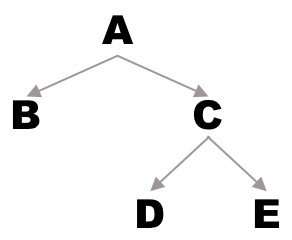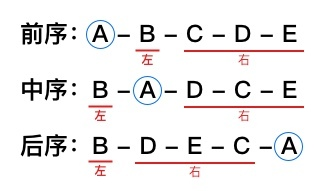# 二叉树深度优先遍历### 深度优先遍历### 前中后序特征

• 前序的第一个一定是 root 节点，后序的最后一个一定是 root 节点

• 每种排序的左子树和右子树分布都是有规律的

• 对于每一个子树都遵循上面两个规律的树### 各种推导

• 给定二叉树得出其前/中/后序遍历的序列；

• 根据前序和中序推导后序（或者推导整颗二叉树）；

• 根据后序和中序推导前序（或者推导整颗二叉树）；

public void recur(int level, int param) {	    // terminator    if (level > MAX_LEVEL) {        // process result     	return;       }        // process current logic    process(level, param);        // drill down    recur(level+1, newParam);        // restore current status}

int[] preSequence = {1, 2, 3, 4, 5, 6, 7, 8, 9};int[] inSequence = {2, 3, 1, 6, 7, 8, 5, 9, 4};

List<Integer> result = new ArrayList<>();preAndInToPost(0, 0, preSequence.length, preSequence, inSequence, result);

if (length == 0) {	return;}

int i = 0;while (inSequence[inIndex + i] != preSequence[preIndex]) {    i++;}

preAndInToPost(preIndex + 1, inIndex, i, preSequence, inSequence, result);preAndInToPost(preIndex + i + 1, inIndex + i + 1, length - i - 1, preSequence, inSequence, result);result.add(preSequence[preIndex]);

/** * 根据前序和中序推导后序 * * @param preIndex    前序索引 * @param inIndex     中序索引 * @param length      序列长度 * @param preSequence 前序序列 * @param inSequence  中序序列 * @param result      结果序列 */private void preAndInToPost(int preIndex, int inIndex, int length, int[] preSequence, int[] inSequence, List<Integer> result) {    if (length == 0) {        return;    }    int i = 0;    while (inSequence[inIndex + i] != preSequence[preIndex]) {        i++;    }    preAndInToPost(preIndex + 1, inIndex, i, preSequence, inSequence, result);    preAndInToPost(preIndex + i + 1, inIndex + i + 1, length - i - 1, preSequence, inSequence, result);    result.add(preSequence[preIndex]);}

### 参考链接## 评论